Download Presentation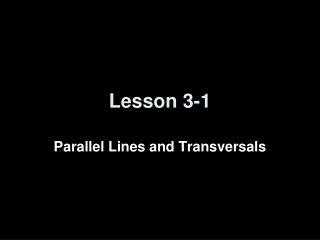Lesson 3-1

# Lesson 3-1 - PowerPoint PPT Presentation

Lesson 3-1. Parallel Lines and Transversals. Transparency 3-1. 5-Minute Check on Chapter 2. 1. Make a conjecture about the next item in the sequence: 5, 20, 80, 320. 2. Write the contrapositive for this statement: If you live in Boston, then you live in Massachusetts.I am the owner, or an agent authorized to act on behalf of the owner, of the copyrighted work described.
Download Presentation## Lesson 3-1

An Image/Link below is provided (as is) to download presentation

Download Policy: Content on the Website is provided to you AS IS for your information and personal use and may not be sold / licensed / shared on other websites without getting consent from its author.While downloading, if for some reason you are not able to download a presentation, the publisher may have deleted the file from their server.

- - - - - - - - - - - - - - - - - - - - - - - - - - E N D - - - - - - - - - - - - - - - - - - - - - - - - - -
Presentation Transcript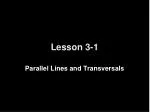### Lesson 3-1

Parallel Lines and Transversals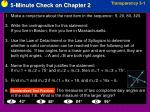Transparency 3-1

5-Minute Check on Chapter 2

1. Make a conjecture about the next item in the sequence: 5, 20, 80, 320.

2. Write the contrapositive for this statement:

If you live in Boston, then you live in Massachusetts.

3. Use the Law of Detachment or the Law of Syllogism to determine whether a valid conclusion can be reached from the following set of statements: If two angles form a linear pair and are congruent, they are both right angles. A and B are both right angles.

4. Name the property that justifies the statement.

If m1 + m2 = 75 and m2 = m3, then m1 + m3 = 75.

5. Find m1 and m2 if m1 = 8x + 18, and m2 = 16x – 6.

6. The measures of two complementary angles are in the ratio 7:8. What is the measure of the larger angle?

Standardized Test Practice:

96

A

42

B

C

84

D

48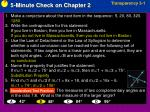Transparency 3-1

5-Minute Check on Chapter 2

1. Make a conjecture about the next item in the sequence: 5, 20, 80, 320.

1280

2. Write the contrapositive for this statement:

If you live in Boston, then you live in Massachusetts.

If you do not live in Massachusetts, then you do not live in Boston.

3. Use the Law of Detachment or the Law of Syllogism to determine whether a valid conclusion can be reached from the following set of statements: If two angles form a linear pair and are congruent, they are both right angles. A and B are both right angles.

no conclusion

4. Name the property that justifies the statement.

If m1 + m2 = 75 and m2 = m3, then m1 + m3 = 75.

Substitution Property

5. Find m1 and m2 if m1 = 8x + 18, and m2 = 16x – 6.

m1 = 74; m2 = 106

6. The measures of two complementary angles are in the ratio 7:8. What is the measure of the larger angle?

Standardized Test Practice:

96

A

42

B

C

84

D

48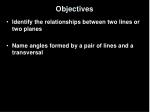Objectives
• Identify the relationships between two lines or two planes
• Name angles formed by a pair of lines and a transversal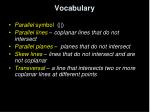Vocabulary
• Parallel symbol (||)
• Parallel lines – coplanar lines that do not intersect
• Parallel planes – planes that do not intersect
• Skew lines – lines that do not intersect and are not coplanar
• Transversal – a line that intersects two or more coplanar lines at different points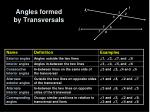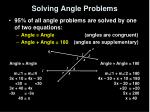t

k

1

2

3

4

5

6

l

7

8

Solving Angle Problems
• 95% of all angle problems are solved by one of two equations:
• Angle = Angle (angles are congruent)
• Angle + Angle = 180 (angles are supplementary)

Angle = Angle

m1 = m4

3x + 10 = 4x – 30

+30 = +30

3x + 40 = 4x

-3x = -3x

40 = x

Angle + Angle = 180

m1 + m2 = 180

4x – 30 + x + 10 = 180

5x – 20 = 180

+20 = +20

5x = 200

x = 40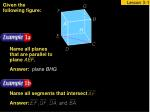Name all segments that intersect

Given the following figure:

Name all planes that are parallel to plane AEF.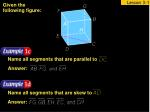Name all segments that are parallel to

Name all segments that are skew to

Given the following figure: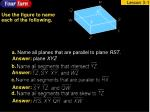a. Name all planes that are parallel to plane RST.

b.

c.

d.

Use the figure to name each of the following.BUS STATION Some of a bus station’s driveways are shown as lines u, v, w, x, y and z.

Identify the sets of lines to which line vis a transversal.

Answer: If the lines are extended, line v intersects lines u, w, x, and z.

Identify the sets of lines to which line yis a transversal.

Answer: lines u, w, x, zBUS STATION Some of a bus station’s driveways are shown as lines u, v, w, x, y and z.

Identify the sets of lines to which line uis a transversal.

Answer: lines v, x, y, z

Identify the sets of lines to which line wis a transversal.

Answer: lines v, x, y, za. line a

b. line b

c. line c

d. line d

HIKING A group of nature trails is shown. Identify the sets of lines to which each given line is a transversal.

Answer: lines c, d, e, f

Answer: lines c, d, e, f

Answer: lines a, b, d, e, f

Answer: lines a, b, c, e, fGiven the following figure, identify each set of angles as alternate interior, alternate exterior, corresponding, or consecutive interiorangles.d.

a.

b.

e.

c.

f.

Identify each set of angles as alternate interior, alternate exterior, corresponding, or consecutive interiorangles.